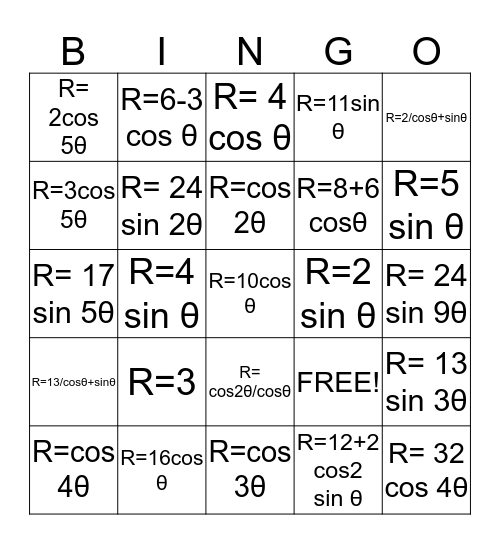# trig BingoThis bingo card has 25 words: R=3, R=cos 3θ, R=cos 2θ, R=cos 4θ, R=2/cosθ+sinθ, R= 2cos 5θ, R=3cos 5θ, R=6-3 cos θ, R=2 sin θ, R= cos2θ/cosθ, R=8+6 cosθ, R= 17 sin 5θ, FREE!, R= 13 sin 3θ, R= 24 sin 2θ, R= 32 cos 4θ, R= 24 sin 9θ, R= 4 cos θ, R=5 sin θ, R=4 sin θ, R=10cos θ, R=16cos θ, R=13/cosθ+sinθ, R=11sin θ and R=12+2 cos2 sin θ.

## Play Online

Share this URL with your players:For more control of your online game, create a clone of this card first.

## Probabilities

With players vying for a you'll have to call about __ items before someone wins. There's a __% chance that a lucky player would win after calling __ items.

Tip: If you want your game to last longer (on average), add more unique words/images to it.